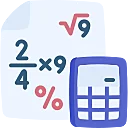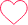# Divisibility Tips, Tricks and Shortcuts

## Tips and Tricks for Divisibility

Divisibility is a mathematical concept that deals with the relationship between numbers and how one number can be evenly divided by another. When we say that one number is divisible by another, it means that the division operation results in a whole number or an integer without any remainder.### Tips, Tricks and Divisibility Rules

• Divisibility rule for 1
• Every number is divisible by 1.
Example: 5 is divisible by 1
• Divisibility rule for 2
• Any even number or number whose last digit is an even number (0, 2, 4, 6, 8) is divisible by
Example: 220 is divisible by 2.

• Divisibility rule for 3
• A number is divisible by 3 if the sum of its digits is divisible by 3.
Example: 315 is divisible by 3.
Here, 3 + 1 + 5 = 9

9 is divisible by 3. It means 315 is also divisible by 3.

• Divisibility rule for 4
• A number is divisible by 4, if the number formed by the last two digits is divisible by 4.
Example: 7568 is divisible by 4
Here, 68 is divisible by 4 (68÷4 = 17)

Therefore, 7568 is divisible by 4

• Divisibility rule for 5
• A number is exactly divisible by 5 if it has the digits 0 or 5 at one’s place.
Example: 5900, 57895, 4400, 1010 are divisible by 5.
• Divisibility rule for 6
• A number is exactly divisible by 6 if that number is divisible by 2 and 3 both. It is because 2 and 3 are prime factors of 6.
Example: 63894 is divisible by 6, the last digit is 4, so divisible by 2, and sum 6+3+8+9+4 = 30 is divisible by 3.
• Divisibility rule for 7
• Double the last digit and subtract it from the remaining leading truncated number to check if the result is divisible by 7 until no further division is possible
Example: 1093 is divisible by 7

Remove 3 from the number and double it = 6

Remaining number is 109, now subtract 6 from 109 = 109 – 6 = 103.

Repeat the process, We have last digit as 3, double = 6

Remaining number is 10, now subtract 6 from 10 = 10 – 6 = 4.

As 4 is not divisible by 7, hence the number 1093 is not divisible by 7.

• Divisibility rule for 8
• If the last three digits of a number are divisible by 8, then the number is completely divisible by 8.

Example: 215632 is divisible by 8, as last three digits 632 is divisible by 8.

• Divisibility rule for 9
• It is the same as of divisibility of 3. Sum of digits in the given number must be divisible by 9.
Example: 312768 is divisible by 9, Sum of digits = 3+1+2+7+6+8 = 27 is divisible by 9.
• Divisibility rule for 10
• Any number whose last digit is 0, is divisible by 10.
Example: 10, 60, 370, 1000, etc.
• Divisibility rule for 11
• If the difference of the sum of alternative digits of a number is divisible by 11, then that number is divisible by 11.
Example: 737 is divisible by 11 as 7 + 7 = 14 and 14 – 3 = 11, 11 is divisible by 11.
416042 is divisible by 11 as 4 + 6 + 4 = 14 and 1 + 0+ 2 = 3, 14 – 3 = 11, 11 is divisible by 11.
• Divisibility rule for 12
• A number is exactly divisible by 12 if that number is divisible by 3 and 4 both.
Example: 108 is divisible by 12. Sum of digit = 1 + 8 = 9, 9 is divisible by 3. And last two
digits 08 is divisible by 4. Therefore, 108 is divisible by 12.
• Divisibility rule for 13
• Multiply the last digit with 4 and add it to remaining number in a given number, the result must be divisible by 13.
Example: 208 is divisible by 13, 20 + (4 x 8) = 20 + 32 = 52, 52 is divisible by 13.
• Divisibility rule for 14
• A number is exactly divisible by 14 if that number is divisible by 2 and 7 both. It is because 2 and 7 are prime factors of 14.
Example: 1246 is divisible by 14, as the last digit is even, so divisible by 2.
Now check for 7,

Remove 6 from the number and double it = 12

Remaining number is 124, now subtract 124 from 12 = 112.

Repeat the process, We have the last digit as 2, double = 4

The remaining number is, now subtract 11 from 4 = 7

As 7 is divisible by 7, hence the number 1246 is divisible by 7.

• Divisibility rule for 15
• If the number divisible by both 3 and 5, it is divisible by 15.
• Example: 23505 is divisible by 15.
• Check for 3: 2 + 3 + 5 + 0 +5 = 15, 15 is divisible by 3.
Check for 5: It has the 5 at one’s place, therefore, divisible by 5.
• Divisibility rule for 16
• The number formed by last four digits in the given number must be divisible by 16.
Example: 152448 is divisible by 16 as last four digits (2448) are divisible by 16.
• Divisibility rule for 17
• Multiply the last digit with 5 and subtract it from remaining number in a given number, the
result must be divisible by 17.
Example: 136 is divisible by 17. 13 – (5 x 6) = 13 – 30 = 17, 17 is divisible by 17.
• Divisibility rule for 18
• If the number is divisible by both 2 and 9, it is divisible by 18.
Example: 92754 is divisible by 18.
Check for 2: the last digit is even, therefore, it is divisible by 2.
Check for 9: 9 + 2 + 7 + 5 + 4 = 27, 27 is divisible by 9.
• Divisibility rule for 19
• Multiply the last digit with 2 and add it to remaining number in a given number, the result must be divisible by 19.
Example: 285 is divisible by 19,
28 + (2 x 5) = 28 + 10 = 38, 38 is divisible by 19.
• Divisibility rule for 20
• The number formed by last two digits in the given number must be divisible by 20.
Example: 245680 is divisible by 20, because the last two digits 80 is divisible by 20.

### Related Banners

Get PrepInsta Prime & get Access to all 200+ courses offered by PrepInsta in One Subscription

### Type 1: Find the largest or smallest number

Question 1. Find the smallest 4 digit number which is exactly divisible by 41?

Options.

A. 1000

B. 1023

C. 1025

D. 1012

Solution     Smallest 4 digit number is 1000

On dividing 1000 by 41, remainder = 16

Required number = 1000 + (41 – 16) = 1025

Correct option: C

Question 2. Find the Largest 3 digit number which is exactly divisible by 25?

Options.

A. 975

B. 905

C. 980

D. 950

Solution     Largest Three digit numbers is 999
On dividing 999 by 25, remainder = 24
Required number = 999 – 24 = 975
Correct option: A

### Type 2: Which of the following numbers is/or not divisible by given number.

Question 1. Which of these numbers is divisible by 3?

Options.

A. 1003

B.  253

C. 1031

D. 1221

Solution     1003 = 1 + 0 + 0 + 3 = 4, 4 is not divisible by 3

253 = 2 + 5 + 3 = 10, 10 is not divisible by 3

1031 = 1 + 0 + 3 + 1 = 5, 5 is not divisible by 3

1221 = 1 + 2 + 2 + 1= 6, 6 is divisible by 3

Correct option: D

Question 2. Which of these numbers is not divisible by 10?

Options.

A. 1250

B. 1253

C. 1930

D. 1220

Solution      Last digit of 1253  is not 0 so it is not divisible by 10

Correct option: B

### Type 3: Tips and Tricks to Solve Divisibility Questions.Find the remainder

Question 1. Find out the remainder of $\frac{2^{12}}{5}$

Options.

A. 1

B. 2

C. 0

D. 3

Solution     Convert $2^{12}$ in multiple of 16  = 16 x 16 x 16
=$2^{4}\times 2^{4}\times 2^{4}$

Now divide each number by 5

On dividing 16 by 5 we get remainder as 1

Now, multiply all the remainders 1 x 1 x 1 = 1

Correct option: A

Question 2. Find out the remainder when $7^{4}$ is divided by 5.

Options.

A. 0

B. 4

C. 1

D. 2

Solution    Divide 7 by 5 Remainder will 2

$2\times 2\times 2\times 2$ = 16

Now divide 16 by 5

On dividing 16 by 5 we get remainder as 1

Correct option: C

## Get over 200+ course One Subscription

Courses like AI/ML, Cloud Computing, Ethical Hacking, C, C++, Java, Python, DSA (All Languages), Competitive Coding (All Languages), TCS, Infosys, Wipro, Amazon, DBMS, SQL and others

## Checkout list of all the video courses in PrepInsta Prime Subscription

### 7 comments on “Divisibility Tips, Tricks and Shortcuts”

•Subhransu Surya

In Type 1:Find the largest or smallest number, if it ask for largest how to proceed3
•Subhransu Surya

I got it.4
•PrepInsta

Contact the mentors on our telegram support group where teachers will answer you accordingly.1
•Gudla

where we can find mock tests5
•HelpPrepInsta

Hey Gudla, which companies mock tests are you looking for ??0
•Anwesh

Help me out in the last question? I don’t understand3
•HelpPrepInsta

Hello Anwesh,2